# Statistics review 10: Further nonparametric methods

## Abstract

This review introduces nonparametric methods for testing differences between more than two groups or treatments. Three of the more common tests are described in detail, together with multiple comparison procedures for identifying specific differences between pairs of groups.

## Introduction

The previous review in this series  described analysis of variance, the method used to test for differences between more than two groups or treatments. However, in order to use analysis of variance, the observations are assumed to have been selected from Normally distributed populations with equal variance. The tests described in this review require only limited assumptions about the data.

The Kruskal–Wallis test is the nonparametric alternative to one-way analysis of variance, which is used to test for differences between more than two populations when the samples are independent. The Jonckheere–Terpstra test is a variation that can be used when the treatments are ordered. When the samples are related, the Friedman test can be used.

## Kruskal–Wallis test

The Kruskal–Wallis test is an extension of the Mann–Whitney test  for more than two independent samples. It is the nonparametric alternative to one-way analysis of variance. Instead of comparing population means, this method compares population mean ranks (i.e. medians). For this test the null hypothesis is that the population medians are equal, versus the alternative that there is a difference between at least two of them.

The test statistic for one-way analysis of variance is calculated as the ratio of the treatment sum of squares to the residual sum of squares . The Kruskal–Wallis test uses the same method but, as with many nonparametric tests, the ranks of the data are used in place of the raw data.

This results in the following test statistic: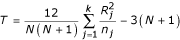Where Rj is the total of the ranks for the jth sample, nj is the sample size for the jth sample, k is the number of samples, and N is the total sample size, given by: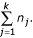This is approximately distributed as a χ2 distribution with k - 1 degrees of freedom. Where there are ties within the data set the adjusted test statistic is calculated as: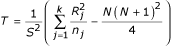Where rij is the rank for the ith observation in the jth sample, nj is the number of observations in the jth sample, and S2 is given by the following: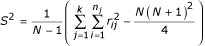For example, consider the length of stay following admission to three intensive care units (ICUs): cardiothoracic, medical and neurosurgical. The data in Table 1 show the length of stay of a random sample of patients from each of the three ICUs. As with the Mann–Whitney test, the data must be ranked as though they come from a single sample, ignoring the ward. Where two values are tied (i.e. identical), each is given the mean of their ranks. For example, the two 7s each receive a rank of (5 + 6)/2 = 5.5, and the three 11s a rank of (9 +10 + 11)/3 = 10. The ranks are shown in brackets in Table 2.

For the data in Table 1, the sums of ranks for each ward are 29.5, 48.5 and 75, respectively, and the total sum of the squares of the individual ranks is 5.52 + 12 + ... + 102 = 1782.5. The test statistic is calculated as follows: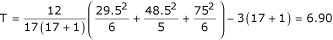This gives a P value of 0.032 when compared with a χ2 distribution with 2 degrees of freedom. This indicates a significant difference in length of stay between at least two of the wards. The test statistic adjusted for ties is calculated as follows: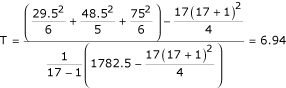This gives a P value of 0.031. As can be seen, there is very little difference between the unadjusted and the adjusted test statistics because the number of ties is relatively small. This test is found in most statistical packages and the output from one is given in Table 3.

### Multiple comparisons

If the null hypothesis of no difference between treatments is rejected, then it is possible to identify which pairs of treatments differ by calculating a least significant difference. Treatments i and j are significantly different at the 5% significance level if the difference between their mean ranks is greater than the least significant difference (i.e. if the following inequality is true):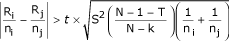Where t is the value from the t distribution for a 5% significance level and N - k degrees of freedom.

For the data given in Table 1, the least significant difference when comparing the cardiothoracic with medical ICU, or medical with neurosurgical ICU, and the difference between the mean ranks for the cardiothoracic and medical ICUs are as follows: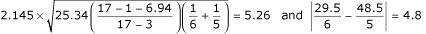The difference between the mean ranks for the cardiothoracic and medical ICUs is 4.8, which is less than 5.26, suggesting that the average length of stay in these ICUs does not differ. The same conclusion can be reached when comparing the medical with neurosurgical ICU, where the difference between mean ranks is 4.9. However, the difference between the mean ranks for the cardiothoracic and neurosurgical ICUs is 7.6, with a least significant difference of 5.0 (calculated using the formula above with ni = nj = 6), indicating a significant difference between length of stays on these ICUs.

## The Jonckheere–Terpstra test

There are situations in which treatments are ordered in some way, for example the increasing dosages of a drug. In these cases a test with the more specific alternative hypothesis that the population medians are ordered in a particular direction may be required. For example, the alternative hypothesis could be as follows: population median1 ≤ population median2 ≤ population median3. This is a one-tail test, and reversing the inequalities gives an analagous test in the opposite tail. Here, the Jonckheere–Terpstra test can be used, with test statistic TJT calculated as: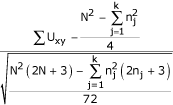Where Uxy is the number of observations in group y that are greater than each observation in group x. This is compared with a standard Normal distribution.

This test will be illustrated using the data in Table 1 with the alternative hypothesis that time spent by patients in the three ICUs increases in the order cardiothoracic (ICU 1), medical (ICU 2) and neurosurgical (ICU 3).

U12 compares the observations in ICU 1 with ICU 2. It is calculated as follows. The first value in sample 1 is 7; in sample 2 there are three higher values and a tied value, giving 7 the score of 3.5. The second value in sample 1 is 1; in sample 2 there are 5 higher values giving 1 the score of 5. U12 is given by the total scores for each value in sample 1: 3.5 + 5 + 5 + 4 + 2.5 + 3 = 23. In the same way U13 is calculated as 6 + 6 + 6 + 6 + 4.5 + 6 = 34.5 and U23 as 6 + 6 + 2 + 4.5 + 1 = 19.5. Comparisons are made between all combinations of ordered pairs of groups. For the data in Table 1 the test statistic is calculated as follows: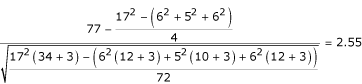Comparing this with a standard Normal distribution gives a P value of 0.005, indicating that the increase in length of stay with ICU is significant, in the order cardiothoracic, medical and neurosurgical.

## The Friedman Test

The Friedman test is an extension of the sign test for matched pairs  and is used when the data arise from more than two related samples. For example, the data in Table 4 are the pain scores measured on a visual–analogue scale between 0 and 100 of five patients with chronic pain who were given four treatments in a random order (with washout periods). The scores for each patient are ranked. Table 5 contains the ranks for Table 4. The ranks replace the observations, and the total of the ranks for each patient is the same, automatically removing differences between patients.

In general, the patients form the blocks in the experiment, producing related observations. Denoting the number of treatments by k, the number of patients (blocks) by b, and the sum of the ranks for each treatment by R1, R2 ... Rk, the usual form of the Friedman statistic is as follows: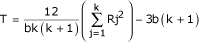Under the null hypothesis of no differences between treatments, the test statistic approximately follows a χ2 distribution with k - 1 degrees of freedom. For the data in Table 4: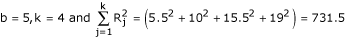This gives the following: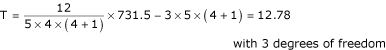Comparing this result with tables, or using a computer package, gives a P value of 0.005, indicating there is a significant difference between treatments.

An adjustment for ties is often made to the calculation. The adjustment employs a correction factor C = (bk [k + 1]2)/4. Denoting the rank of each individual observation by rij, the adjusted test statistic is: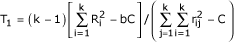For the data in Table 4: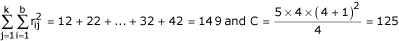Therefore, T1 = 3 × [731.5-5 × 125]/(149 - 125) = 13.31, giving a smaller P value of 0.004.

### Multiple comparisons

If the null hypothesis of no difference between treatments is rejected, then it is again possible to identify which pairs of treatments differ by calculating a least significant difference. Treatments i and j are significantly different at the 5% significance level if the difference between the sum of their ranks is more than the least significant difference (i.e. the following inequality is true):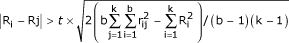Where t is the value from the t distribution for a 5% significance level and (b - 1)(k - 1) degrees of freedom.

For the data given in Table 4, the degrees of freedom for the least significant difference are 4 × 3 = 12 and the least significant difference is: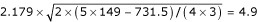The difference between the sum of the ranks for treatments B and C is 5.5, which is greater than 4.9, indicating that these two treatments are significantly different. However, the difference in the sum of ranks between treatments A and B is 4.5, and between C and D it is 3.5, and so these pairs of treatments have not been shown to differ.

## Limitations

The advantages and disadvantages of nonparametric methods were discussed in Statistics review 6 . Although the range of nonparametric tests is increasing, they are not all found in standard statistical packages. However, the tests described in the present review are commonly available.

When the assumptions for analysis of variance are not tenable, the corresponding nonparametric tests, as well as being appropriate, can be more powerful.

## Conclusion

The Kruskal–Wallis, Jonckheere–Terpstra and Friedman tests can be used to test for differences between more than two groups or treatments when the assumptions for analysis of variance are not held.

Further details on the methods discussed in this review, and on other nonparametric methods, can be found, for example, in Sprent and Smeeton  or Conover .

## Abbreviations

ICU:

intensive care unit.

## References

1. Bewick V, Cheek L, Ball J: Statistics review 9: Analysis of variance. Crit Care. 2004, 7: 451-459. 10.1186/cc2401.

2. Whitely E, Ball J: Statistics review 6: Nonparametric methods. Crit Care. 2002, 6: 509-513. 10.1186/cc1820.

3. Sprent P, Smeeton NC: Applied Nonparametric Statistical Methods. 2001, London, UK: Chapman & Hall/CRC, 3

4. Conover WJ: Practical Nonparametric Statistics. 1999, New York, USA: John Wiley & Sons, 3

## Author information

Authors

### Corresponding author

Correspondence to Viv Bewick.

None declared.

## Rights and permissions

Reprints and Permissions

Bewick, V., Cheek, L. & Ball, J. Statistics review 10: Further nonparametric methods. Crit Care 8, 196 (2004). https://doi.org/10.1186/cc2857